Anzeige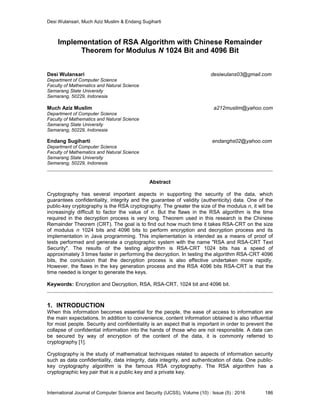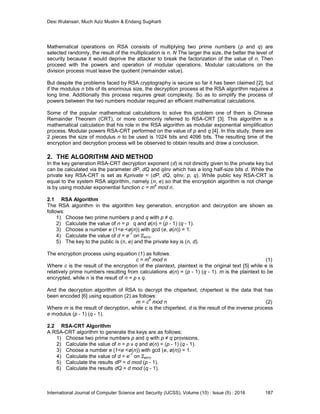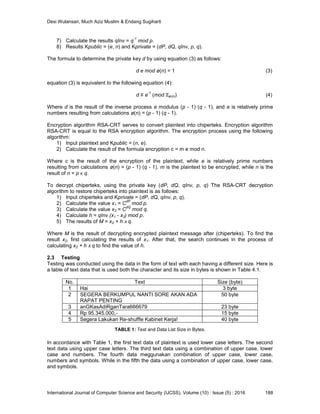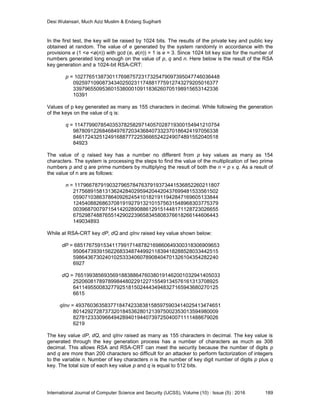Anzeige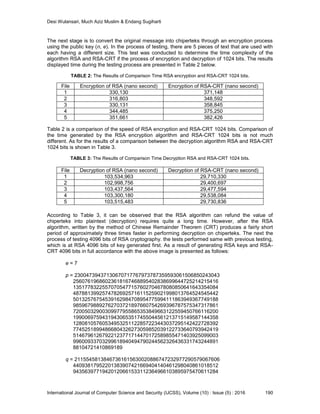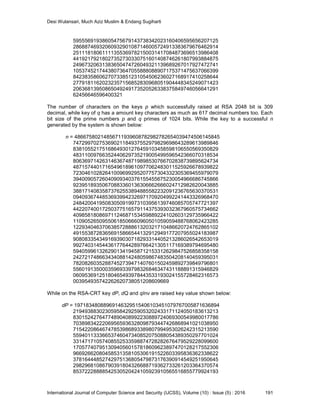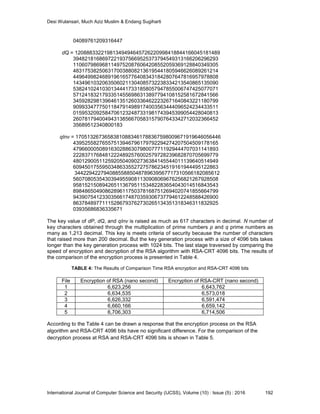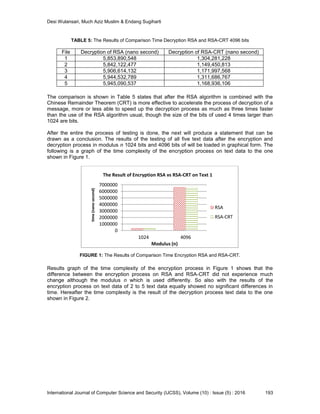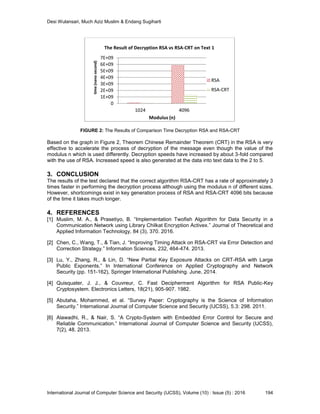Nächste SlideShare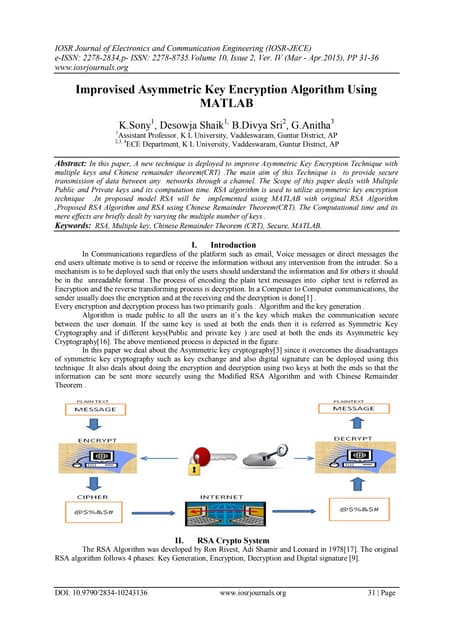F010243136
1 von 9
Anzeige

### Implementation of RSA Algorithm with Chinese Remainder Theorem for Modulus N 1024 Bit and 4096 Bit

1. Desi Wulansari, Much Aziz Muslim & Endang Sugiharti International Journal of Computer Science and Security (IJCSS), Volume (10) : Issue (5) : 2016 186 Implementation of RSA Algorithm with Chinese Remainder Theorem for Modulus N 1024 Bit and 4096 Bit Desi Wulansari desiwulans03@gmail.com Department of Computer Science Faculty of Mathematics and Natural Science Semarang State University Semarang, 50229, Indonesia Much Aziz Muslim a212muslim@yahoo.com Department of Computer Science Faculty of Mathematics and Natural Science Semarang State University Semarang, 50229, Indonesia Endang Sugiharti endanghs02@yahoo.com Department of Computer Science Faculty of Mathematics and Natural Science Semarang State University Semarang, 50229, Indonesia Abstract Cryptography has several important aspects in supporting the security of the data, which guarantees confidentiality, integrity and the guarantee of validity (authenticity) data. One of the public-key cryptography is the RSA cryptography. The greater the size of the modulus n, it will be increasingly difficult to factor the value of n. But the flaws in the RSA algorithm is the time required in the decryption process is very long. Theorem used in this research is the Chinese Remainder Theorem (CRT). The goal is to find out how much time it takes RSA-CRT on the size of modulus n 1024 bits and 4096 bits to perform encryption and decryption process and its implementation in Java programming. This implementation is intended as a means of proof of tests performed and generate a cryptographic system with the name "RSA and RSA-CRT Text Security". The results of the testing algorithm is RSA-CRT 1024 bits has a speed of approximately 3 times faster in performing the decryption. In testing the algorithm RSA-CRT 4096 bits, the conclusion that the decryption process is also effective undertaken more rapidly. However, the flaws in the key generation process and the RSA 4096 bits RSA-CRT is that the time needed is longer to generate the keys. Keywords: Encryption and Decryption, RSA, RSA-CRT, 1024 bit and 4096 bit. 1. INTRODUCTION When this information becomes essential for the people, the ease of access to information are the main expectations. In addition to convenience, content information obtained is also influential for most people. Security and confidentiality is an aspect that is important in order to prevent the collapse of confidential information into the hands of those who are not responsible. A data can be secured by way of encryption of the content of the data, it is commonly referred to cryptography . Cryptography is the study of mathematical techniques related to aspects of information security such as data confidentiality, data integrity, data integrity, and authentication of data. One public- key cryptography algorithm is the famous RSA cryptography. The RSA algorithm has a cryptographic key pair that is a public key and a private key.
2. Desi Wulansari, Much Aziz Muslim & Endang Sugiharti International Journal of Computer Science and Security (IJCSS), Volume (10) : Issue (5) : 2016 187 Mathematical operations on RSA consists of multiplying two prime numbers (p and q) are selected randomly, the result of the multiplication is n. N The larger the size, the better the level of security because it would deprive the attacker to break the factorization of the value of n. Then proceed with the powers and operation of modular operations. Modular calculations on the division process must leave the quotient (remainder value). But despite the problems faced by RSA cryptography is secure so far it has been claimed , but if the modulus n bits of its enormous size, the decryption process at the RSA algorithm requires a long time. Additionally this process requires great complexity. So as to simplify the process of powers between the two numbers modular required an efficient mathematical calculations. Some of the popular mathematical calculations to solve this problem one of them is Chinese Remainder Theorem (CRT), or more commonly referred to RSA-CRT . This algorithm is a mathematical calculation that his role in the RSA algorithm as modular exponential simplification process. Modular powers RSA-CRT performed on the value of p and q . In this study, there are 2 pieces the size of modulus n to be used is 1024 bits and 4096 bits. The resulting time of the encryption and decryption process will be observed to obtain results and draw a conclusion. 2. THE ALGORITHM AND METHOD In the key generation RSA-CRT decryption exponent (d) is not directly given to the private key but can be calculated via the parameter dP, dQ and qInv which has a long half-size bits d. While the private key RSA-CRT is set as Kprivate = (dP, dQ, qInv, p, q). While public key RSA-CRT is equal to the system RSA algorithm, namely (n, e) so that the encryption algorithm is not change is by using modular exponential function c = m e mod n. 2.1 RSA Algorithm The RSA algorithm in the algorithm key generation, encryption and decryption are shown as follows: 1) Choose two prime numbers p and q with p ≠ q. 2) Calculate the value of n = p q and ø(n) = (p - 1) (q - 1). 3) Choose a number e (1<e <ø(n)) with gcd (e, ø(n)) = 1. 4) Calculate the value of d = e -1 on ℤø(n). 5) The key to the public is (n, e) and the private key is (n, d). The encryption process using equation (1) as follows: c = m e mod n (1) Where c is the result of the encryption of the plaintext, plaintext is the original text  while e is relatively prime numbers resulting from calculations ø(n) = (p - 1) (q - 1). m is the plaintext to be encrypted, while n is the result of n = p q. And the decryption algorithm of RSA to decrypt the chipertext, chipertext is the data that has been encoded  using equation (2) as follows: m = c d mod n (2) Where m is the result of decryption, while c is the chipertext. d is the result of the inverse process e modulus (p - 1) (q - 1). 2.2 RSA-CRT Algorithm A RSA-CRT algorithm to generate the keys are as follows: 1) Choose two prime numbers p and q with p ≠ q provisions. 2) Calculate the value of n = p q and ø(n) = (p - 1) (q - 1). 3) Choose a number e (1<e <ø(n)) with gcd (e, ø(n)) = 1. 4) Calculate the value of d = e -1 on ℤø(n). 5) Calculate the results dP = d mod (p - 1). 6) Calculate the results dQ = d mod (q - 1).
3. Desi Wulansari, Much Aziz Muslim & Endang Sugiharti International Journal of Computer Science and Security (IJCSS), Volume (10) : Issue (5) : 2016 188 7) Calculate the results qInv = q -1 mod p. 8) Results Kpublic = (e, n) and Kprivate = (dP, dQ, qInv, p, q). The formula to determine the private key d by using equation (3) as follows: d e mod ø(n) = 1 (3) equation (3) is equivalent to the following equation (4): d ≡ e -1 (mod ℤø(n)) (4) Where d is the result of the inverse process e modulus (p - 1) (q - 1), and e is relatively prime numbers resulting from calculations ø(n) = (p - 1) (q - 1). Encryption algorithm RSA-CRT serves to convert plaintext into chiperteks. Encryption algorithm RSA-CRT is equal to the RSA encryption algorithm. The encryption process using the following algorithm: 1) Input plaintext and Kpublic = (n, e). 2) Calculate the result of the formula encryption c = m e mod n. Where c is the result of the encryption of the plaintext, while e is relatively prime numbers resulting from calculations ø(n) = (p - 1) (q - 1). m is the plaintext to be encrypted, while n is the result of n = p q. To decrypt chiperteks, using the private key (dP, dQ, qInv, p, q) The RSA-CRT decryption algorithm to restore chiperteks into plaintext is as follows: 1) Input chiperteks and Kprivate = (dP, dQ, qInv, p, q). 2) Calculate the value x1 = C dP mod p. 3) Calculate the value x2 = C dQ mod q. 4) Calculate h = qInv (x1 - x2) mod p. 5) The results of M = x2 + h q. Where M is the result of decrypting encrypted plaintext message after (chiperteks). To find the result x2, first calculating the results of x1. After that, the search continues in the process of calculating x2 + h q to find the value of h. 2.3 Testing Testing was conducted using the data in the form of text with each having a different size. Here is a table of text data that is used both the character and its size in bytes is shown in Table 4.1. No. Text Size (byte) 1 Hai 3 byte 2 SEGERA BERKUMPUL NANTI SORE AKAN ADA RAPAT PENTING 50 byte 3 anGKasAdiRganTara666679 23 byte 4 Rp 95.345.000,- 15 byte 5 Segera Lakukan Re-shuffle Kabinet Kerja! 40 byte TABLE 1: Text and Data List Size in Bytes. In accordance with Table 1, the first text data of plaintext is used lower case letters. The second text data using upper case letters. The third text data using a combination of upper case, lower case and numbers. The fourth data meggunakan combination of upper case, lower case, numbers and symbols. While in the fifth the data using a combination of upper case, lower case, and symbols.
4. Desi Wulansari, Much Aziz Muslim & Endang Sugiharti International Journal of Computer Science and Security (IJCSS), Volume (10) : Issue (5) : 2016 189 In the first test, the key will be raised by 1024 bits. The results of the private key and public key obtained at random. The value of e generated by the system randomly in accordance with the provisions e (1 <e <ø(n)) with gcd (e, ø(n)) = 1 is e = 3. Since 1024 bit key size for the number of numbers generated long enough on the value of p, q and n. Here below is the result of the RSA key generation and a 1024-bit RSA-CRT: p = 10277651387301176987572317325479097395047746036448 09259710908734340250231174881775912743279205016377 33979655095360153800010911836260705198915653142336 10391 Values of p key generated as many as 155 characters in decimal. While following the generation of the keys on the value of q is: q = 11477990785403537825829714057028719300154941210754 98780912268468497672034368407332370186424197056338 84617243251249168877722536665242249074891552040518 84923 The value of q raised key has a number no different from p key values as many as 154 characters. The system is processing the steps to find the value of the multiplication of two prime numbers p and q are prime numbers by multiplying the result of both the n = p q. As a result of the value of n are as follows: n = 11796678791903279657847637919373441536852260211807 21756891581313624284029594204420437699481533561502 05907103863786409262454101821911942847169605133844 12454088268637081919279132101575631548968303775379 00396870079715414202890886129151448171128723026655 67529874887655142902239658345808376618266144606443 149034893 While at RSA-CRT key dP, dQ and qInv raised key value shown below: dP = 68517675915341179917148782169860649300318306909653 95064739391562268334874499211839418288528033442515 59864367302401025333406078908404701326104354282240 6927 dQ = 76519938569356918838864760380191462001032941405033 25206081789789984480229122715549134576161313708925 64114955008327792518150244434948327165943680270125 6615 qInv = 49376036358377184742338381585975903414025413474651 80142927287373201845362801213975002353013594980009 82781233309664942894019440739725040071111488679026 6219 The key value dP, dQ, and qInv raised as many as 155 characters in decimal. The key value is generated through the key generation process has a number of characters as much as 308 decimal. This allows RSA and RSA-CRT can meet the security because the number of digits p and q are more than 200 characters so difficult for an attacker to perform factorization of integers to the variable n. Number of key characters n is the number of key digit number of digits p plus q key. The total size of each key value p and q is equal to 512 bits.
5. Desi Wulansari, Much Aziz Muslim & Endang Sugiharti International Journal of Computer Science and Security (IJCSS), Volume (10) : Issue (5) : 2016 190 The next stage is to convert the original message into chiperteks through an encryption process using the public key (n, e). In the process of testing, there are 5 pieces of text that are used with each having a different size. This test was conducted to determine the time complexity of the algorithm RSA and RSA-CRT if the process of encryption and decryption of 1024 bits. The results displayed time during the testing process are presented in Table 2 below. TABLE 2: The Results of Comparison Time RSA encryption and RSA-CRT 1024 bits. Table 2 is a comparison of the speed of RSA encryption and RSA-CRT 1024 bits. Comparison of the time generated by the RSA encryption algorithm and RSA-CRT 1024 bits is not much different. As for the results of a comparison between the decryption algorithm RSA and RSA-CRT 1024 bits is shown in Table 3. TABLE 3: The Results of Comparison Time Decryption RSA and RSA-CRT 1024 bits. According to Table 3, it can be observed that the RSA algorithm can refund the value of chiperteks into plaintext (decryption) requires quite a long time. However, after the RSA algorithm, written by the method of Chinese Remainder Theorem (CRT) produces a fairly short period of approximately three times faster in performing decryption on chiperteks. The next the process of testing 4096 bits of RSA cryptography. the tests performed same with previous testing, which is at RSA 4096 bits of key generated first. As a result of generating RSA keys and RSA- CRT 4096 bits in full accordance with the above image is presented as follows: e = 7 p = 23004739437130670717767973787359593061506850243043 25607619686023618167468895402838699644725214215416 13517783225570705477157602704678080850641643354084 48788139925747826925716115259021998013764524545442 50132576754539162984708954775994111863949367749188 98596798892762703721897660754269396787575347317861 72005032900309977955865353849663122559450766116200 19900697594319430653517455044561213715149587144358 12808105760534953251122857223443037295142422728392 77452518994866804326273059852039122733640793942419 51467961267922123771714470172589855471403925099003 99600933703299618940494790244562326436331743244891 88104721410869189 q = 21155458138467361615630020886747232977290579067606 44093817952201383907421669404140461298040861018512 94356397719420120661533112364966103895975470611284 File Encryption of RSA (nano second) Encryption of RSA-CRT (nano second) 1 330,130 371,148 2 316,803 348,592 3 330,131 358,845 4 344,485 375,250 5 351,661 382,426 File Decryption of RSA (nano second) Decryption of RSA-CRT (nano second) 1 103,534,963 29,710,330 2 102,998,756 29,400,697 3 103,437,564 29,477,594 4 103,300,180 29,538,084 5 103,515,483 29,730,836
6. Desi Wulansari, Much Aziz Muslim & Endang Sugiharti International Journal of Computer Science and Security (IJCSS), Volume (10) : Issue (5) : 2016 191 59555691938605475679143738342023160406595656207125 28688746932060932901087146005724913383679676462914 25111818061111355369782150031417084873696513986408 44192179218027352730330751601408746261807993884875 24967320631383650474726049321139689267017927472741 10537452174438073647055888088907175371475637066399 84238358606270733851231054506236027168917410258644 27791811620232357156852830968051904448345249071423 20636813950865049249173520526338375849746056641291 62456646596400321 The number of characters on the keys p which successfully raised at RSA 2048 bit is 309 decimal, while key of q has a amount key characters as much as 617 decimal numbers too. Each bit size of the prime numbers p and q primes of 1024 bits. While the key to a successful n generated by the system is shown below: n = 48667580214856711939608782982782654039474506145845 74729970275369021184937552979829698643289613989846 83810552175168649301276459103458598106550569350829 48311009766352440629735219005499596542366070318534 80636971426314636748719898530766702838739895624734 48715744017165496189610977062483011525926678939822 72304610282641009699295207757304332305369455979079 39400905726040909340376155455675230054966686745866 92395189350670883360136306662666024712982620043885 38817140835873762553894885582232091236765630370531 09409367448536939942326971709204992241443326968470 24842004195083050919973103956139746085705747721397 44220740017250377516579114375393032367960575734662 40985818086971124687153459889224102603129735966422 11090526509550618506660960501059059488768062423285 12293404637063857288861320321710486620724762865102 49155387283656915866544132912949177207955024183987 90808335434916939030718293314405213286026542653019 99274031454436177644289766421305117169389794695480 59405996132629013419458712153312629847526858358158 24272174866343408814248059867483504208140459395031 78208260352887452739471407601502459892739849796801 55601913500035969339798326846347431188891315946829 06095369125180465493978443533193024155728462316573 003954935742262620738051208609669 While on the RSA-CRT key dP, dQ and qInv are raised key value shown below: dP = 19718348088969146329515406103451079767005871636894 21949388302305958429259053202433171124050183613213 83015242764774890408992230889724069300549980017786 70389834222069565936328098793447426868941021038950 71542208646747853986893389807994953026242315213590 55940113336653746047340852075088054389350297701024 33147171057408552533598874728282676479529228099600 17057740795130940560157818609623897470128217552306 96692662080458531358105306191522603395836362338622 37816444852742975136805479873176390914549251950645 29829681086790391804326688719362733261203364370574 85372228888542530520424105923910565516855779924193
7. Desi Wulansari, Much Aziz Muslim & Endang Sugiharti International Journal of Computer Science and Security (IJCSS), Volume (10) : Issue (5) : 2016 192 04089761209316447 dQ = 12088833221981349494645726220998418844166045181489 39482181686972219375669525373794549313166206296293 11060798696811497520876064208552059369128840349305 48317538250631700388082136195441805946626089261214 44964998246891961657764083431842807647816957978808 14349610320635060211304085732238334213540865135090 53824102410301344417331858057947855006747425077071 57124183217933514556986313897794108152581672841566 34592829813964613512603364622232671640843221180799 90993347775011847914989174003563444096524234433511 01595320925847061232487331981743945399054428040813 26078179400494313856670583157907643342712032366452 35689512340800183 qInv = 17051326736583810883461788367598009671919646056446 43952558276557513946796179792294274207504509178165 47966000508916302886307980077711929444707031141893 22283717684812224892576002579728239682870705699779 48012900511259205040902736384145544011139640514949 60945017559503486335527275786234519161944495122863 34422942279408855885048789639567717310566182085612 56070805354303949559081130908069676256821267928508 95815215089426511367951153482283654043014516843543 89848650490862896117503781687512694020741855664799 94390754123303566174870359306737794612248588426900 86378489771115286793762730265134351318346311832925 03935686836335671 The key value of dP, dQ, and qInv is raised as much as 617 characters in decimal. N number of key characters obtained through the multiplication of prime numbers p and q prime numbers as many as 1,213 decimal. This key is meets criteria of security because the number of characters that raised more than 200 decimal. But the key generation process with a size of 4096 bits takes longer than the key generation process with 1024 bits. The last stage traversed by comparing the speed of encryption and decryption of the RSA algorithm with RSA-CRT 4096 bits. The results of the comparison of the encryption process is presented in Table 4. TABLE 4: The Results of Comparison Time RSA encryption and RSA-CRT 4096 bits According to the Table 4 can be drawn a response that the encryption process on the RSA algorithm and RSA-CRT 4096 bits have no significant difference. For the comparison of the decryption process at RSA and RSA-CRT 4096 bits is shown in Table 5. File Encryption of RSA (nano second) Encryption of RSA-CRT (nano second) 1 6,623,256 6,643,762 2 6,634,535 6,573,018 3 6,626,332 6,591,474 4 6,660,166 6,659,142 5 6,706,303 6,714,506
8. Desi Wulansari, Much Aziz Muslim & Endang Sugiharti International Journal of Computer Science and Security (IJCSS), Volume (10) : Issue (5) : 2016 193 TABLE 5: The Results of Comparison Time Decryption RSA and RSA-CRT 4096 bits The comparison is shown in Table 5 states that after the RSA algorithm is combined with the Chinese Remainder Theorem (CRT) is more effective to accelerate the process of decryption of a message, more or less able to speed up the decryption process as much as three times faster than the use of the RSA algorithm usual, though the size of the bits of used 4 times larger than 1024 are bits. After the entire the process of testing is done, the next will produce a statement that can be drawn as a conclusion. The results of the testing of all five text data after the encryption and decryption process in modulus n 1024 bits and 4096 bits of will be loaded in graphical form. The following is a graph of the time complexity of the encryption process on text data to the one shown in Figure 1. FIGURE 1: The Results of Comparison Time Encryption RSA and RSA-CRT. Results graph of the time complexity of the encryption process in Figure 1 shows that the difference between the encryption process on RSA and RSA-CRT did not experience much change although the modulus n which is used differently. So also with the results of the encryption process on text data of 2 to 5 text data equally showed no significant differences in time. Hereafter the time complexity is the result of the decryption process text data to the one shown in Figure 2. 0 1000000 2000000 3000000 4000000 5000000 6000000 7000000 1024 4096 time(nanosecond) Modulus (n) The Result of Encryption RSA vs RSA-CRT on Text 1 RSA RSA-CRT File Decryption of RSA (nano second) Decryption of RSA-CRT (nano second) 1 5,853,890,548 1,304,281,228 2 5,842,122,477 1,149,450,813 3 5,906,614,132 1,171,997,568 4 5,944,532,789 1,311,686,767 5 5,945,090,537 1,168,936,106
9. Desi Wulansari, Much Aziz Muslim & Endang Sugiharti International Journal of Computer Science and Security (IJCSS), Volume (10) : Issue (5) : 2016 194 FIGURE 2: The Results of Comparison Time Decryption RSA and RSA-CRT Based on the graph in Figure 2, Theorem Chinese Remainder Theorem (CRT) in the RSA is very effective to accelerate the process of decryption of the message even though the value of the modulus n which is used differently. Decryption speeds have increased by about 3-fold compared with the use of RSA. Increased speed is also generated at the data into text data to the 2 to 5. 3. CONCLUSION The results of the test declared that the correct algorithm RSA-CRT has a rate of approximately 3 times faster in performing the decryption process although using the modulus n of different sizes. However, shortcomings exist in key generation process of RSA and RSA-CRT 4096 bits because of the time it takes much longer. 4. REFERENCES  Muslim, M. A., & Prasetiyo, B. “Implementation Twofish Algorithm for Data Security in a Communication Network using Library Chilkat Encryption Activex.” Journal of Theoretical and Applied Information Technology, 84 (3), 370. 2016.  Chen, C., Wang, T., & Tian, J. “Improving Timing Attack on RSA-CRT via Error Detection and Correction Strategy.” Information Sciences, 232, 464-474. 2013.  Lu, Y., Zhang, R., & Lin, D. “New Partial Key Exposure Attacks on CRT-RSA with Large Public Exponents.” In International Conference on Applied Cryptography and Network Security (pp. 151-162), Springer International Publishing. June, 2014.  Quisquater, J. J., & Couvreur, C. Fast Decipherment Algorithm for RSA Public-Key Cryptosystem. Electronics Letters, 18(21), 905-907. 1982.  Abutaha, Mohammed, et al. “Survey Paper: Cryptography is the Science of Information Security.” International Journal of Computer Science and Security (IJCSS), 5.3: 298. 2011.  Alawadhi, R., & Nair, S. “A Crypto-System with Embedded Error Control for Secure and Reliable Communication.” International Journal of Computer Science and Security (IJCSS), 7(2), 48. 2013. 0 1E+09 2E+09 3E+09 4E+09 5E+09 6E+09 7E+09 1024 4096 time(nanosecond) Modulus (n) The Result of Decryption RSA vs RSA-CRT on Text 1 RSA RSA-CRT
Anzeige